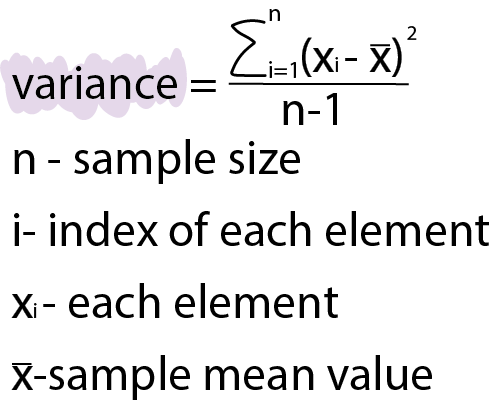Course Content

Probability Theory Basics

## Sample variance

Sample variance is a statistical measure that quantifies the spread or dispersion of a set of data points in a dataset from their mean value.
We can calculate it using the following formula:Note

In this context, sample size and sample mean refer to characteristics calculated based on the existing dataset.

You should keep in mind a fundamental empirical rule: the greater the sample variance, the more spread out the data is.

### How to calculate sample variance in Python?

In NumPy you need to put the sequence of values (in our case, the column of the dataset) into the function `np.var()` like `np.var(df['salary'])` to calculate sample variance.

## Standard deviation

This value is similar to the variance because standard deviation is a square root of the variance.
We can calculate it using `np.std()` function using NumPy library.

Note

All characteristics of datasets are considered in Probability Theory Mastering course in more detail! In this course there is also explored the connection between the concepts of probability theory and the statistical properties of the data, understanding how these concepts are interrelated.

Everything was clear?

Section 6. Chapter 5